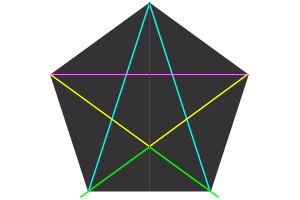# PentagonA regular pentagon has the following dimensions:

AB=BC=CD=DE=AE=BG=EG=1

BF=EF=Φ/2 = cos 36 = sin 54 = $$\frac{1+\sqrt{5}}{4}$$

CG=DG= Φ-1 = 1/Φ = $$\frac{\sqrt5-1}{2}$$

AF=FG= cos 54 = sin 36 = $$\sqrt{\frac{5-\sqrt5}{8}}$$

AG= 2cos 54 = 2sin 36= $$\sqrt{\frac{5-\sqrt5}{2}}$$

CH=DH=BK=CK=1/2

Height AH=EK=$$\frac12\cos 18 = \frac12\sin 72 = \frac12\sqrt{5+2\sqrt5}$$

Circumradius AJ=$$\sqrt{\frac{5+\sqrt5}{10}}$$ = BJ=CJ=DJ=EJ

Inradius JK=HJ= $$\sqrt{\frac{5+2\sqrt5}{20}}$$ = $$\frac{AH}{\sqrt5}$$

FH= cos 18 =sin 72 = $$\sqrt{\frac{5+\sqrt5}{8}}$$

FJ=JK/Φ

GJ=AJ/Φ

GH=AF/Φ=$$\frac12\sqrt{5-2\sqrt5}$$

ABGE is a equilateral rhombus, with angles {108°, 54°, 108°, 54°} and diagonals of length Φ and $$\sqrt{\frac{5-\sqrt5}{2}}$$.

The small pentagon in the center has sides $$1/\Phi^2 = \frac{3-\sqrt5}{2}$$ times that of the larger.